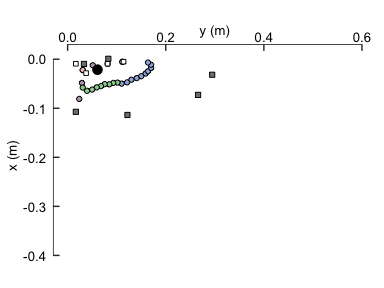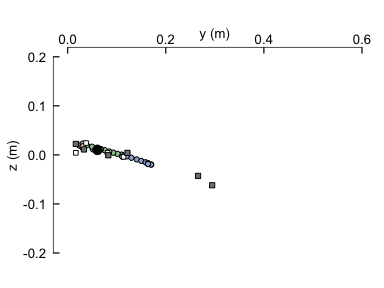# Determine the inertial characteristics of a flying bird.

This package was developed to determine the center of gravity and moment of inertia for a bird in a general flight configuration. This code assumes that birds are a composite structure of simple geometric shapes. For details on the specific assumptions please refer to:

library(AvInertia)

Generally, you should already have all of the necessary measurements loaded into R in the form of a database. We have included the data set from our paper. The bird properties are reported in the metric system and all measurements have been taken with the origin placed at the bird (vehicle) reference point (VRP).

data(dat_id_curr, package = "AvInertia")
data(dat_bird_curr, package = "AvInertia")
data(dat_feat_curr, package = "AvInertia")
data(dat_bone_curr, package = "AvInertia")
data(dat_mat, package = "AvInertia")
data(clean_pts, package = "AvInertia")

## 1. Determine the center of gravity of the bird’s torso (including the legs)

dat_torsotail_out = massprop_restbody(dat_id_curr, dat_bird_curr)

## 2. Calculate the inertia of the flight feathers about the tip of the calamus

feather_inertia <- compute_feat_inertia(dat_mat, dat_feat_curr, dat_bird_curr)

## 3. Determine the center of gravity of one of the bird’s wings

dat_wing_out      = massprop_birdwing(dat_id_curr, dat_bird_curr, dat_bone_curr, dat_feat_curr, dat_mat, clean_pts, feather_inertia, plot_var = 0)

Visualize the center of gravity of each wing component in the x and y axis

dat_wing_out      = massprop_birdwing(dat_id_curr, dat_bird_curr, dat_bone_curr, dat_feat_curr, dat_mat, clean_pts, feather_inertia, plot_var = "yx")or the y and z axis

dat_wing_out      = massprop_birdwing(dat_id_curr, dat_bird_curr, dat_bone_curr, dat_feat_curr, dat_mat, clean_pts, feather_inertia, plot_var = "yz")## 4. If computing for asymmetric case, input a different set of clean points (clean_pts)

Note: that in this case the wing should still be input as it it is on the right-hand side of the bird. The following code will adjust.

## 5. Combine all data and obtain the center of gravity, moment of inertia and principal axes of the bird

curr_full_bird      = combine_inertialprop(dat_torsotail_out,dat_wing_out,dat_wing_out, dat_id_curr, dat_bird_curr, symmetric=TRUE)

This will return a long format data frame with all the individual components I about the VRP and both the full bird I about the VRP and about the full center of gravity.